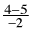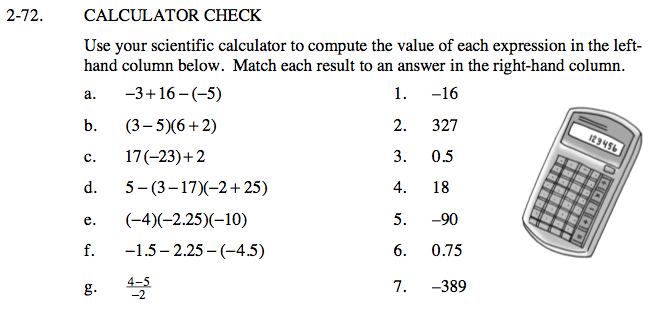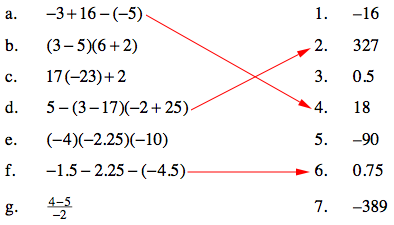### Home > CAAC > Chapter 2 > Lesson 2.1.7 > Problem2-72

2-72.CALCULATOR CHECK

Use your scientific calculator to compute the value of each expression in the left-hand column below. Match each result to an answer in the right-hand column. Homework Help ✎

 a. −3 + 16−(−5) 1. −16 b. (3 − 5)(6 + 2) 2. 327 c. 17(−23) + 2 3. 0.5 d. 5 − (3 − 17)(−2 + 25) 4. 18 e. (−4)(−2.25)(−10) 5. −90 f. −1.5 −2.25 −(−4.5) 6. 0.75 g.7. −389Rewrite the equation using parentheses before putting it into the calculator.

Remember to double check the equation you entered in the calculator to make sure it is the same as the problem.